# Advanced Logic and Conditionals

### Coding 9

Learning about advanced logic and conditional will help you earn points in the technical part of the Demo Video section of the rubric. It will also potentially help you make your mobile app work better!

In this lesson, you will…

• Learn how to write conditional statements that use logic
• Improve your Magic 8 Ball app so that a person can’t ask a question that doesn’t have a question mark in it.

Key Terms

• Logic Operators - allow computers to make decisions based on multiple conditions
• And - a programming operator that will output  true when all input conditions are true
• Or - a programming operator that will output true when at least one of the input conditions are true
• Not - outputs the opposite of the condition input

### Conditions

In the last coding lesson, you learned how to make your app do different things using conditional statements. In this lesson, you will learn about logic operators. Logic operators allow computers to make decisions based on multiple conditions. In App Inventor, these are blocks that need two inputs. There are three major logic operators that you’ll learn in this section: and, or, and not.

and Operator

The and operator will output true when all of the input conditions are true. If any of the input conditions are false, it will output false. Here are all the possible outcomes when using the and operator.True and True = TrueTrue and False = FalseFalse and True = FalseFalse and False = False

Here’s how you can think about using and in a conditional statement: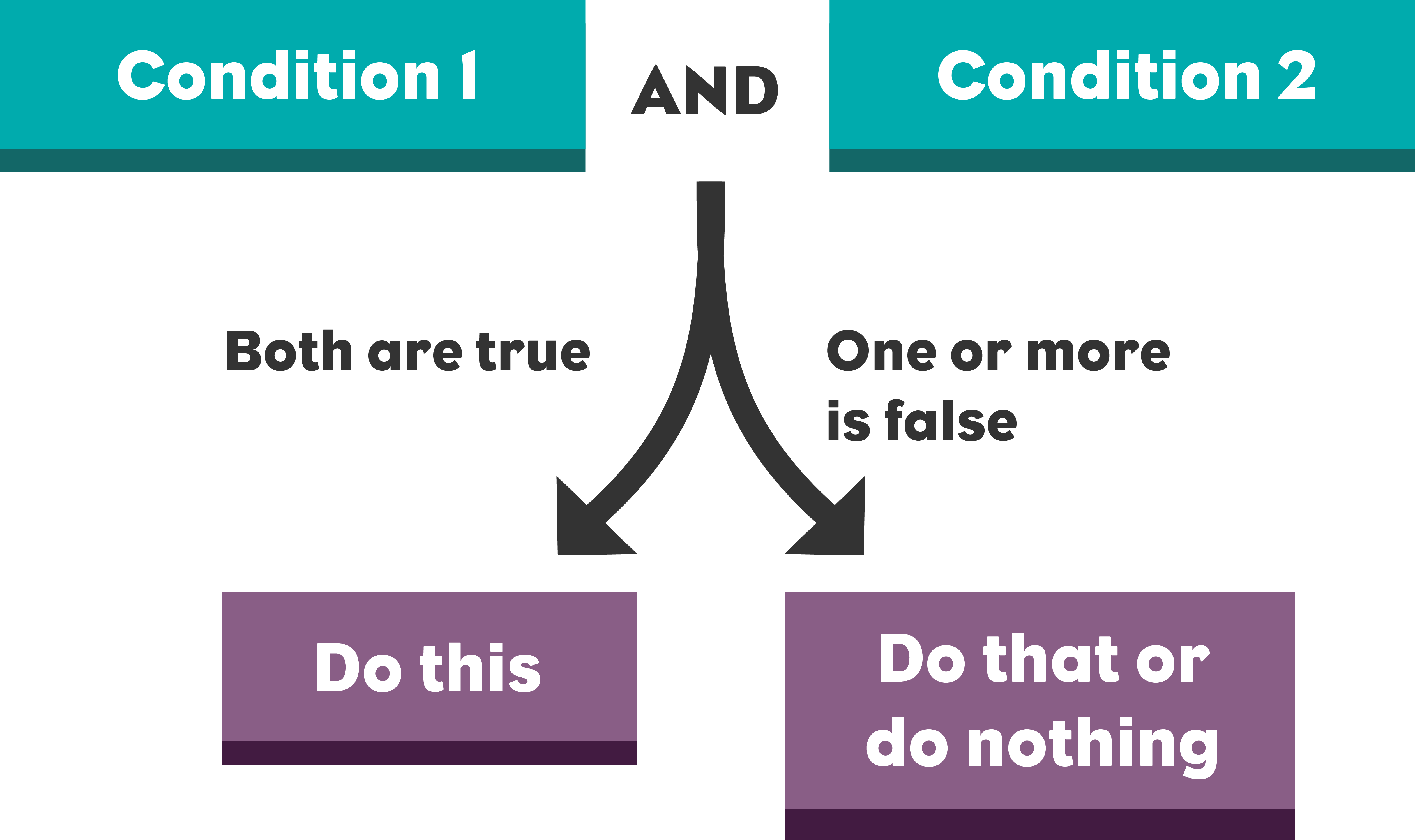Note: Using the and operator is different than using an else if statement because both conditions are evaluated at the same time instead of one after the other.

or Operator

For the or operator to output true, only one of the inputs needs to be true. Here are all the possible outcomes when using the or operator.True or True = TrueTrue or False = TrueFalse or True = TrueFalse or False = False

Here’s how you can think about using or in a conditional statement: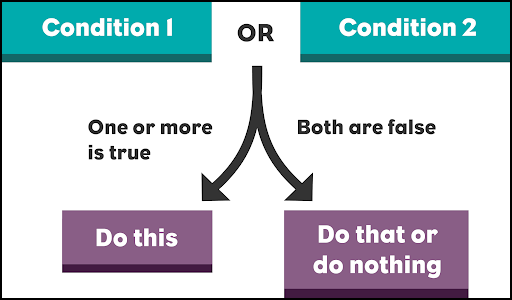Note: The or operator may also seem similar to else if to you. The or operator is different because both conditions are evaluated at the same time instead of one after the other. The or operator is better to use when you have two conditions that should have the same outcome if they are true.

not Operator

The not operator switches the value of an input condition to be the opposite of what it is.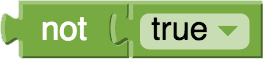not true = falsenot false = true

Here's how you can think of it in a conditional statement.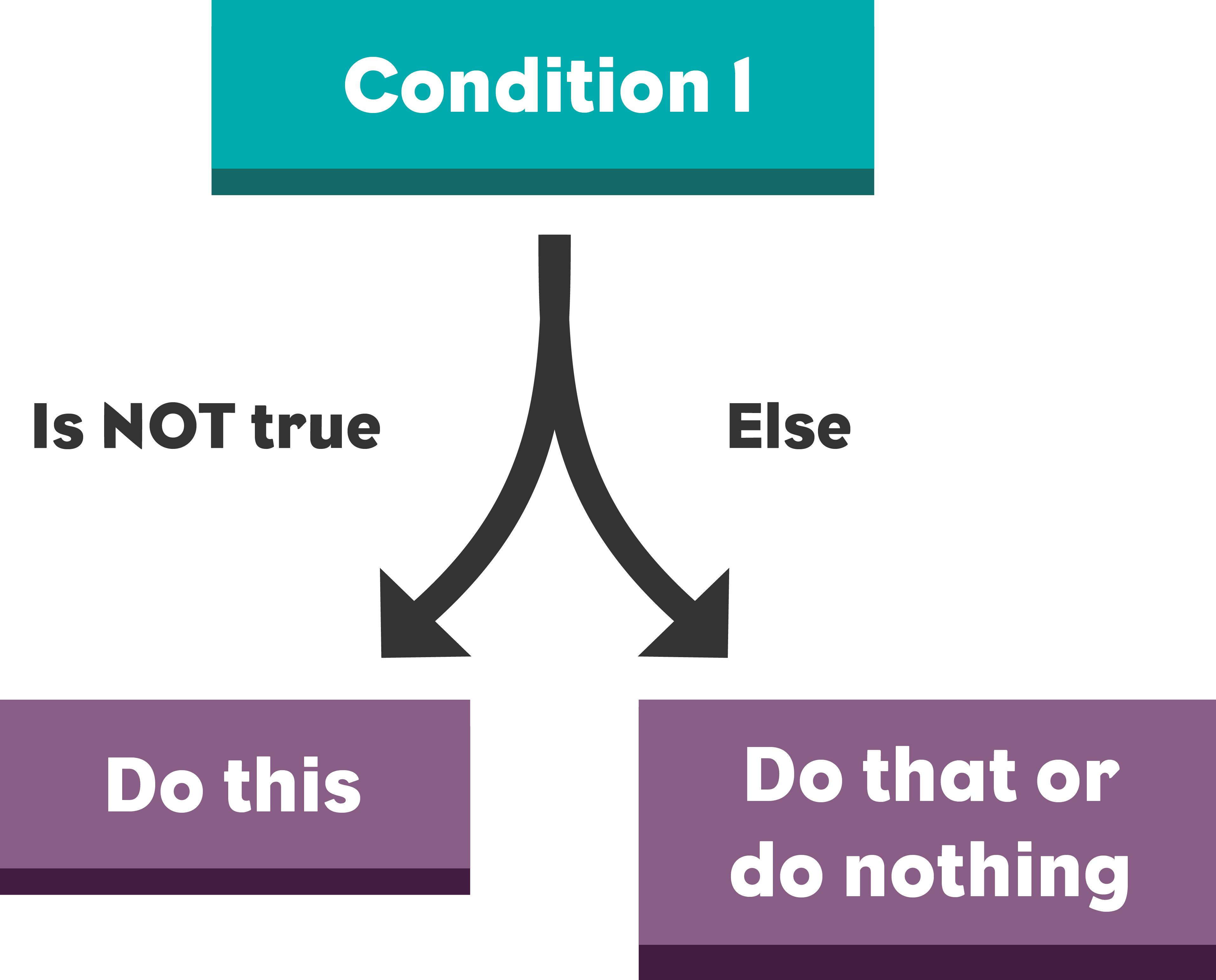### Activity: Magic 8 Ball Improvements - Logic Operators

In this activity, you are going to make one more improvement to your magic 8 ball app using logic operators. Your app will tell users to “ask them a question first” whenever

• The user asks a question that doesn’t have a question mark in it
• The textbox is empty

Question 1: First, what are the two conditions that you need to check for. How could you write these conditions in App Inventor?

Question 2: Do you think you need to use the and operator or the or operator for this? Where do you think this should go in your code?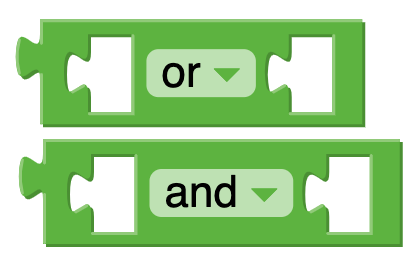Use your Magic 8 Ball code from the past two lessons. If you need the starter code, here it is again:

### Reflection

In this lesson, you learned how to use logic operators to make more advanced conditionals statements.

• What are some other conditions that might be helpful to add to the magic 8 ball app?
• Can you think of some places in your app that you might want to use it?